How to perform PRF photometry?¶

In previous tutorials we discussed how aperture photometry works and how you can create your own custom masks to extract aperture photometry.

As a reminder, aperture photometry works by summing up the flux values across all the pixels in a pre-defined mask. It tends to offer the fastest and most robust way to extract photometry for an isolated star, but it suffers from the three limitations:

1. When two or more stars overlap, aperture photometry will return the sum of the flux of all stars. Separating their signals requires additional assumptions.
2. When the telescope experience motion or focus changes, the fraction of the flux of a star captured by the pre-defined aperture mask will change. This imposes systematics on the data which need to be removed (e.g. using the CBV or SFF techniques).
3. It may be difficult to separate a background signal from the lightcurve of a star. In particular, this is a concern when time-varying background signals are present, such as the so-called "rolling bands" in Kepler.

An alternative to aperture photometry which addresses the above limitations is called called Point Response Function (PRF) model fitting photometry, also known as Point Spread Function (PSF) fitting photometry or PRF (PSF) photometry in short. In this method, a parameterized model is fit to the data. This method is significantly more complicated then aperture photometry and is prone to a different set of systematics. However it offers the ability to separate the signals of overlapping stars in very crowded regions or star clusters.

This tutorial demonstrates how lightkurve can be used to extract lightcurves using the model fitting technique. We will start with an easy example and then show how you can tune the parameters of the model to create more careful analyses.

1. Simple example: PRF photometry of Kepler-10
2. The TPFModel object
3. Visualizing and fitting a TPFModel object
4. Changing TPFModel priors
5. Creating lightcurves with a custom TPFModel object

Simple example: PRF photometry of Kepler-10¶

Let's start with a simple example by obtaining PRF photometry for planet system Kepler-10. We start by downloading the pixel data from the archive:

In :
import lightkurve as lk

The easiest way to get PRF photometry is to simply call the to_lightcurve method with parameter method='prf'. This will fit the pixel data of all cadences with a default model and return the lightcurve.

In :
lc = tpf.to_lightcurve(method='prf')
lc.plot()
Warning: PRF-fitting photometry is experimental in this version of lightkurve.
Fitting cadences: 100%|██████████| 3634/3634 [00:40<00:00, 89.59it/s]
Out:
<matplotlib.axes._subplots.AxesSubplot at 0x1c20b4d940>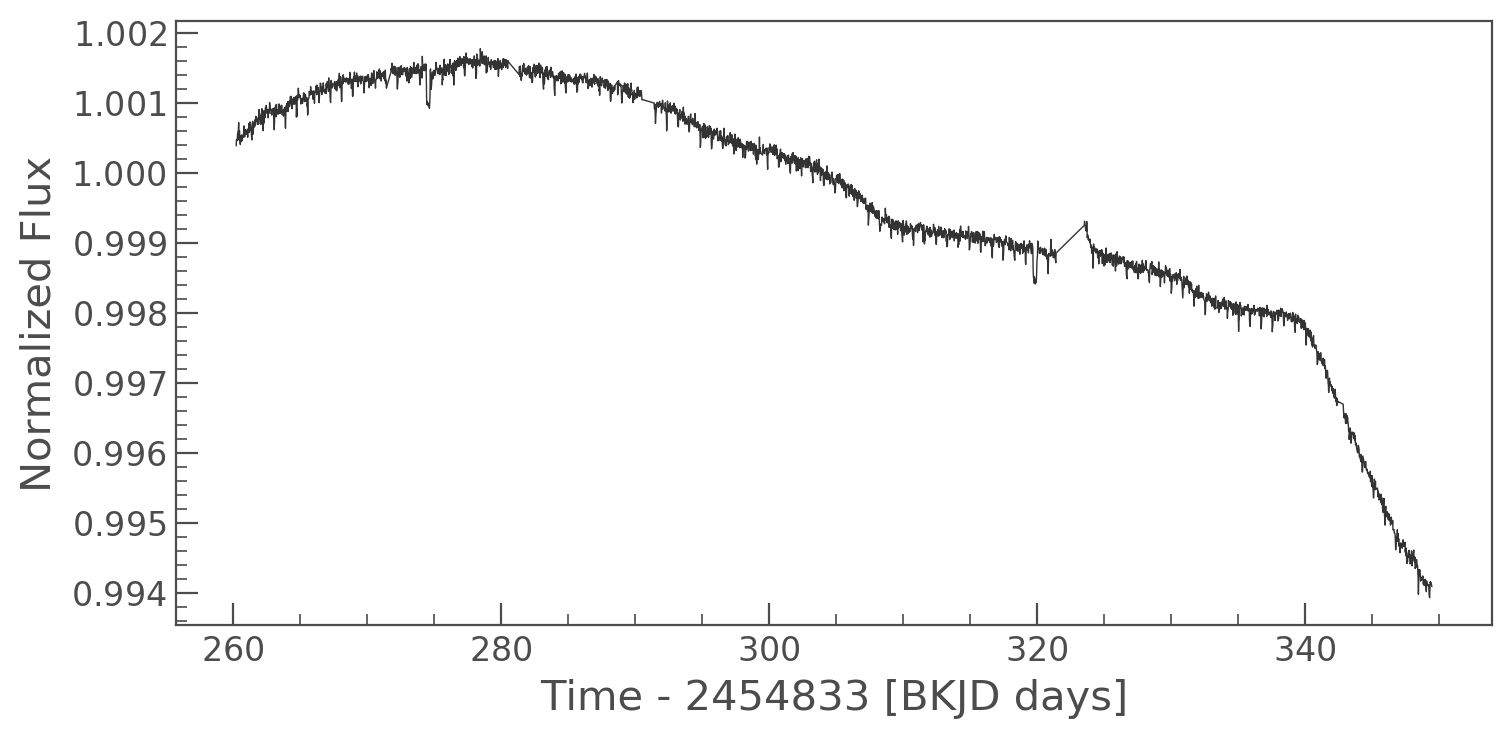A good way to verify that the model-fitting photometry produced a sensible output is by comparing it against standard aperture photometry. You can obtain aperture photometry using the same to_lightcurve method and the parameter method='aperture' (which is the default value of this parameter).

In :
lc_aper = tpf.to_lightcurve(method='aperture')
lc_aper.plot()
Out:
<matplotlib.axes._subplots.AxesSubplot at 0x1c24abf470>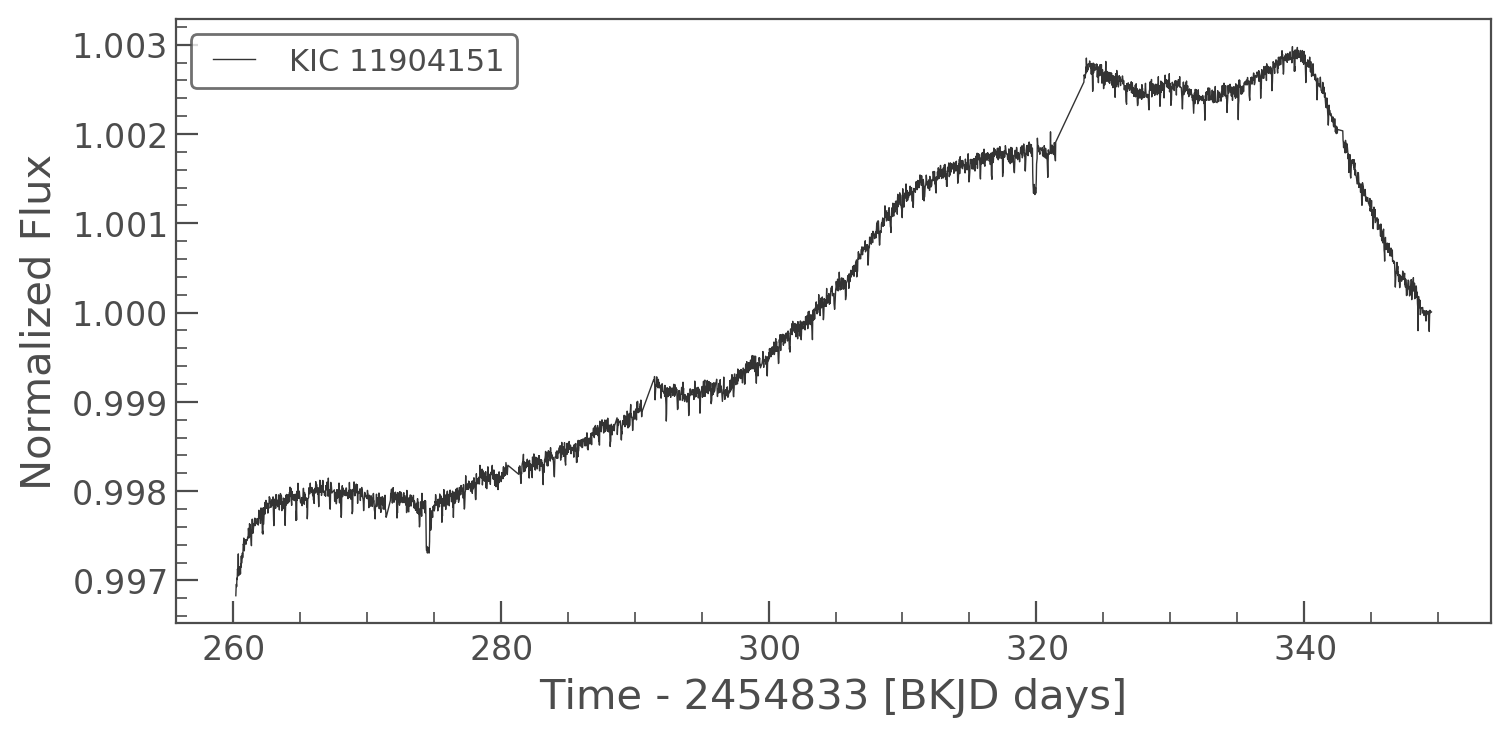There is a notable difference in the long-term trend in both lightcurves. This is because PRF-fitting photometry yields a local estimate of the background which is often different from the Kepler pipeline's global background level estimates.

Let's fold both lightcurves on the period of the known planet Kepler-10 b to show that the transit can be recovered equally well:

In :
import matplotlib.pyplot as plt
_, ax = plt.subplots(nrows=1, ncols=2, figsize=(14,4))
ax.set_title('PRF photometry')
lc.flatten().fold(period=0.8376).plot(ax=ax)
ax.set_title('Aperture photometry')
lc_aper.flatten().fold(period=0.8376).plot(ax=ax)
Out:
<matplotlib.axes._subplots.AxesSubplot at 0x1c23884b70>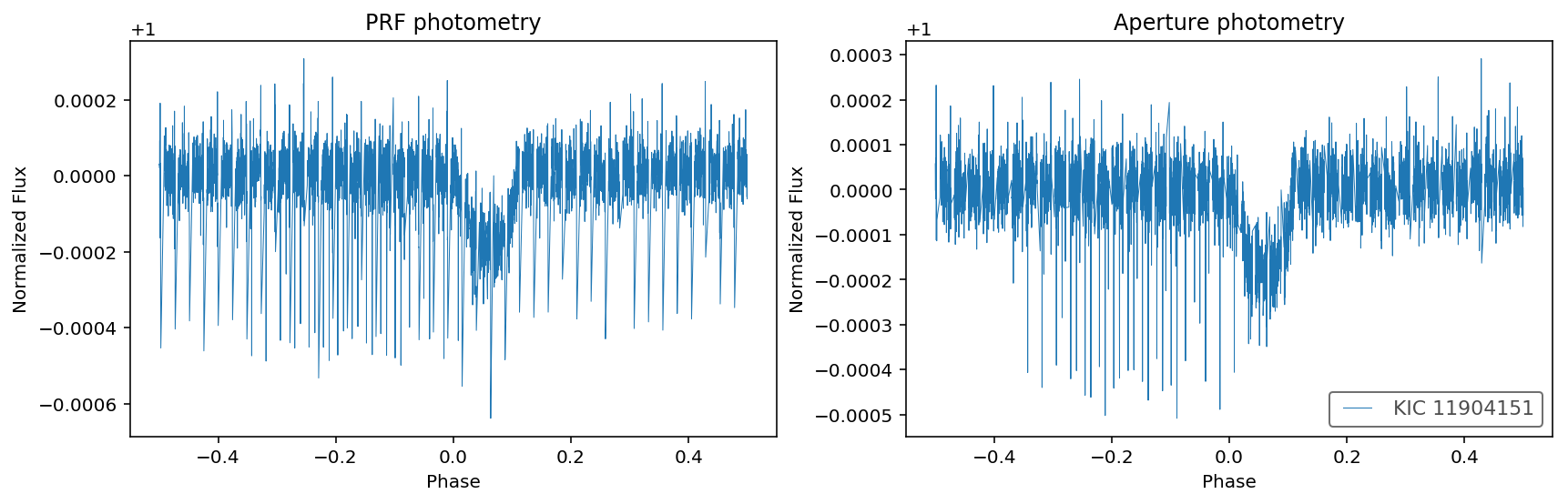Success! Note that the outliers between phase -0.4 and 0.0 are caused by the transits of another planet with a different period.

Note that PRF photometry appears to offer a few percent less noise compared to its aperture counterpart:

In :
print("""The CDPP noise metric equals:
{:.1f} ppm for PRF-fitting photometry;
{:.1f} ppm for aperture photometry.""".format(lc.estimate_cdpp(), lc_aper.estimate_cdpp()))
The CDPP noise metric equals:
48.6 ppm for PRF-fitting photometry;
33.4 ppm for aperture photometry.

This is all you need to know to get started with PRF photometry! In what follows, we explain how you can tune the parameters of the model fitting.

The TPFModel object¶

It is often possible to obtain better results by carefully tuning the model that is being fit to the data. This is true in particular when the data contain multiple overlapping stars. Let's have a look at how you can interact and change the model.

Lightkurve uses objects of type TPFModel to specify the model. You can obtain the default model using the get_model() method:

In :
model = tpf.get_model()
type(model)
Out:
lightkurve.prf.tpfmodel.TPFModel

A TPFModel object encapsulates our beliefs about the stars contained in a pixel file. The key parameters are:

• model.star_priors captures our prior beliefs about the positions and fluxes of the point sources in the data using a list of StarPrior objects. Each StarPrior object is parameterized by col and row in pixel coordinates and flux in electrons/second;
• model.background_prior captures our beliefs about the per-pixel background, parameterized by flux in electrons/second;
• model.focus_prior captures our beliefs on the focus of the spacecraft, parameterized by the unit-less stretch factors scale_col and scale_row, and rotation_angle in radians;
• model.motion_prior captures our beliefs about spacecraft motion, parameterized by shift_col and shift_row in pixel coordinates;
• model.prfmodel an object of type KeplerPRF which models the Point Response Function.
• model.fit_background, model.fit_focus, and model.fit_motion are booleans which indicate whether or not the background, focus, and motion parameters should be considered free parameters, i.e. whether or not our beliefs should assumed to be perfect.

The parameters of each of these components are prior distributions.

We can inspect the our beliefs by accessing the properties, for example, this is the prior on the column pixel coordinate of the star in our data:

In :
model.star_priors.col
Out:
<GaussianPrior(mean=[655.70045778], var=[0.00046541])>

And this is the model's prior on the background flux:

In :
model.background_prior.flux
Out:
<GaussianPrior(mean=[138.52747], var=[145.87004587])>

We can inspect all the parameters at once using the print function:

In :
print(model)
<lightkurve.prf.tpfmodel.TPFModel object at 0x1c238c50b8>
<StarPrior(
col=<GaussianPrior(mean=[655.70045778], var=[0.00046541])>
row=<GaussianPrior(mean=[246.83753465], var=[0.0007464])>
flux=<UniformPrior(lb=[83941.5390625], ub=[1066484.375])>
targetid=11904151)>
<BackgroundPrior(
flux=<GaussianPrior(mean=[138.52747], var=[145.87004587])>)>
<FocusPrior(
scale_col=<GaussianPrior(mean=, var=[0.0001])>
scale_row=<GaussianPrior(mean=, var=[0.0001])>
rotation_angle=<UniformPrior(lb=[-3.1415], ub=[3.1415])>)>
<MotionPrior(
shift_col=<GaussianPrior(mean=, var=[1.])>
shift_row=<GaussianPrior(mean=, var=[1.])>)>
<lightkurve.prf.prfmodel.KeplerPRF object at 0x1c238c5b38>
fit_background=True
fit_focus=False
fit_motion=False

Visualizing and fitting a TPFModel object¶

A TPFModel object contains several methods to interact with the model. For example, you can visualize the default prediction using the plot() method. This will visualize the model prediction using the prior means. Let's plot the model below (left panel) and compare it against the observed data (right panel):

In :
import matplotlib.pyplot as plt
_, ax = plt.subplots(nrows=1, ncols=2, figsize=(14,4))
model.plot(ax=ax)
tpf.plot(ax=ax);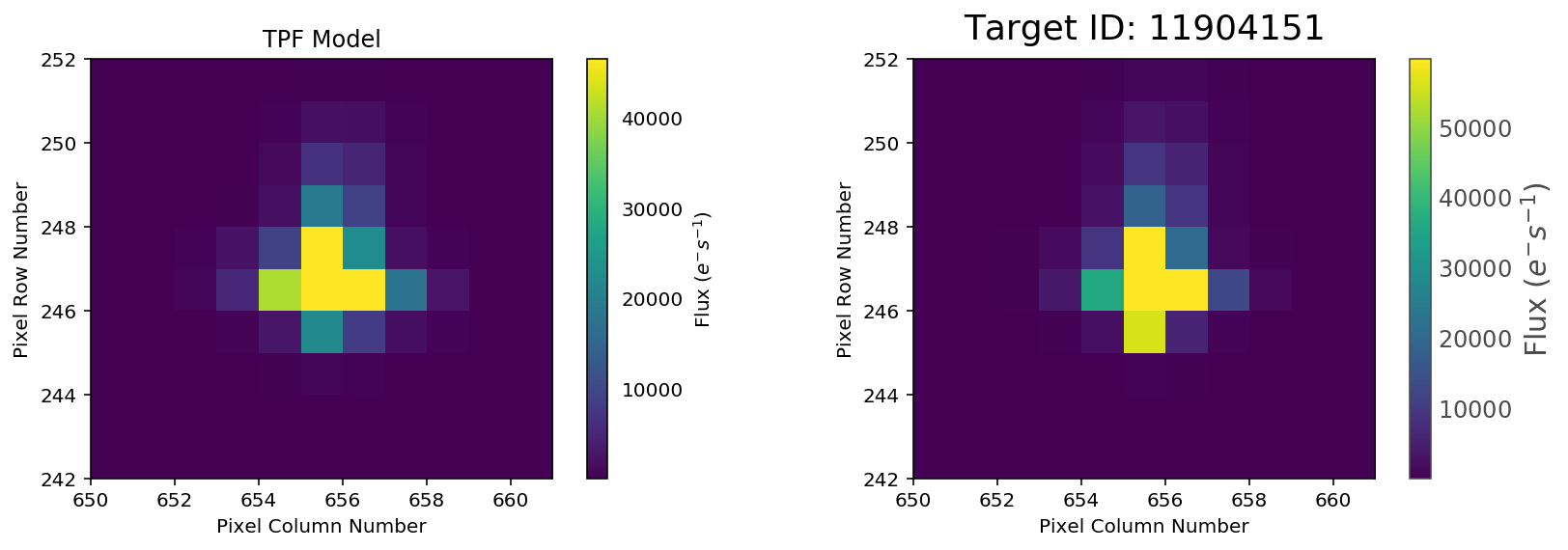You can fit the model to a single cadence of pixel data using the fit() method, which returns an object of type TPFModelParameters which encapulsates the maximum likelihood values of the parameters for that cadence:

In :
parameters = model.fit(tpf.flux + tpf.flux_bkg)
print(parameters)
<lightkurve.prf.tpfmodel.TPFModelParameters object at 0x1c237d66a0>
<StarParameters(
col=655.6409995230879
row=246.75968144624395
flux=528783.6252248927
targetid=None)>
<BackgroundParameters(
flux=175.21544001684765
fitted=True)>
<FocusParameters(
scale_col=
scale_row=
rotation_angle=[0.]
fitted=False)>
<MotionParameters(
shift_col=
shift_row=
fitted=False)>
residual_image:
[ 13.54472234 -24.96701167 -36.46893428 -36.42862056...
predicted_image:
[175.21544002 175.21544002 175.21544002 175.21544002...

For example, we can access the maximum likelihood value of the stellar flux as follows:

In :
parameters.stars.flux
Out:
528783.6252248927

And the background flux was found to be:

In :
parameters.background.flux
Out:
175.21544001684765

We can visualize the model again, this time passing the fitted parameters:

In :
_, ax = plt.subplots(nrows=1, ncols=2, figsize=(12,4))
model.plot(parameters, ax=ax)
tpf.plot(ax=ax)
Out:
<matplotlib.axes._subplots.AxesSubplot at 0x1c236f34a8>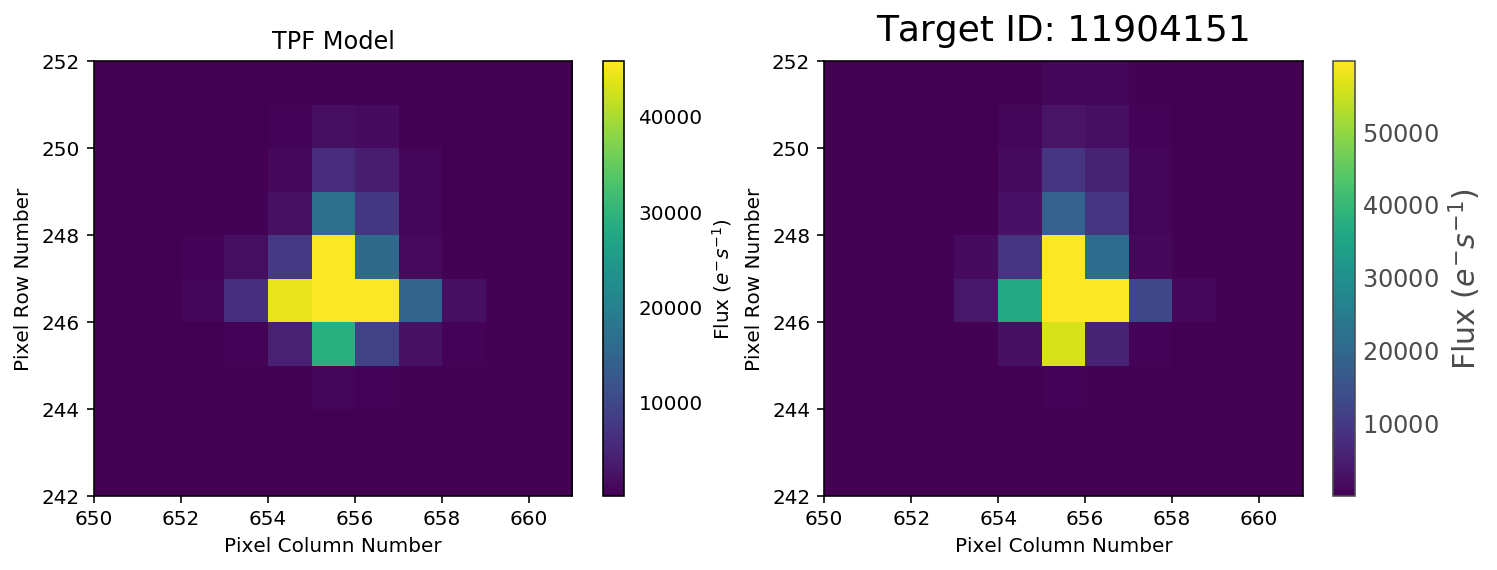Changing TPFModel priors¶

We can change a TPFModel by manipulating its properties. For example, we can add a star to the lower right corner of the pixel file as follows:

In :
from lightkurve.prf import StarPrior
model = tpf.get_model()
model.star_priors.append(StarPrior(col=660, row=243, flux=1e6))
model.plot()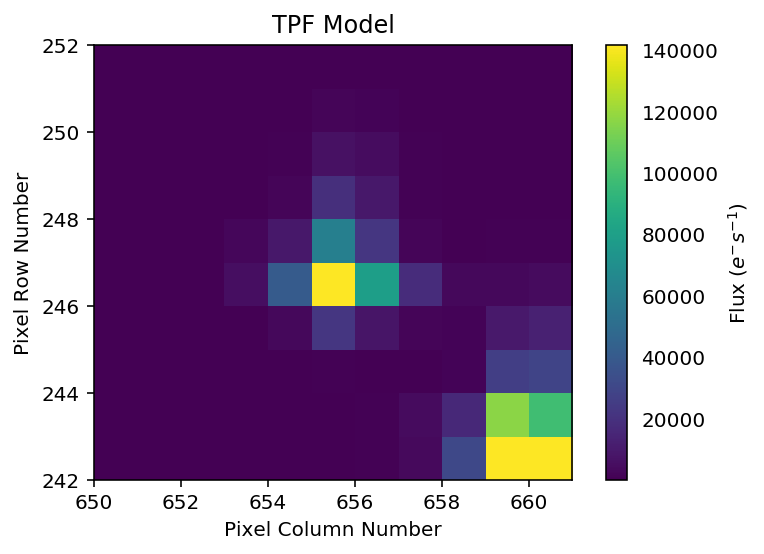Alternatively, you can build a TPFModel from scratch. In the example below, we use an arbitrary combination of Gaussian, Uniform, and Fixed priors to initialize a model. Note that the ideal choices for these assumptions depend strongly on your scientific objectives and may require trial-and-error.

In :
from lightkurve.prf import TPFModel, StarPrior, BackgroundPrior, FocusPrior, MotionPrior
from lightkurve.prf import GaussianPrior, UniformPrior, FixedValuePrior
model = TPFModel(star_priors=[StarPrior(col=GaussianPrior(mean=656, var=1),
row=GaussianPrior(mean=247, var=1),
flux=UniformPrior(lb=0, ub=1e7)),
StarPrior(col=FixedValuePrior(value=660),
row=FixedValuePrior(value=243),
flux=UniformPrior(lb=0, ub=1e7))],
background_prior=BackgroundPrior(flux=GaussianPrior(mean=100., var=10.)),
focus_prior=FocusPrior(scale_col=GaussianPrior(mean=1, var=0.0001),
scale_row=GaussianPrior(mean=1, var=0.0001),
rotation_angle=UniformPrior(lb=-3.1415, ub=3.1415)),
motion_prior=MotionPrior(shift_col=GaussianPrior(mean=0., var=0.01),
shift_row=GaussianPrior(mean=0., var=0.01)),
prfmodel=tpf.get_prf_model(),
fit_background=True,
fit_focus=False,
fit_motion=False)
model.plot()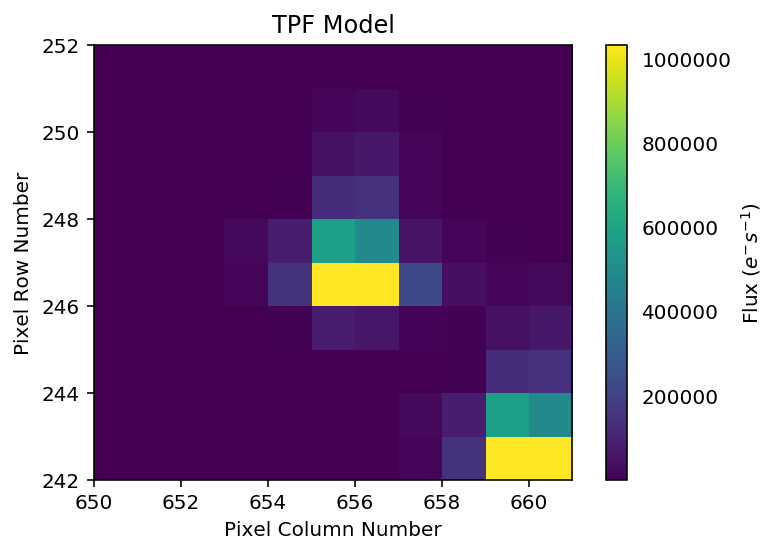Creating lightcurves with a custom TPFModel object¶

Above we demonstrated how you can interact with a TPFModel and fit its parameters to a single cadence. Most users will be interested in obtaining a lightcurve by fitting the model to all the cadences rather than a single one.

To make this easy, lightkurve provides a helper class called PRFPhotometry, which makes it easy to fit the model to all cadences and inspect the results. For example:

In :
from lightkurve import PRFPhotometry
prfphot = PRFPhotometry(model=tpf.get_model())
prfphot.run(tpf.flux + tpf.flux_bkg)
prfphot.plot_results()
Fitting cadences: 100%|██████████| 3634/3634 [00:40<00:00, 89.26it/s]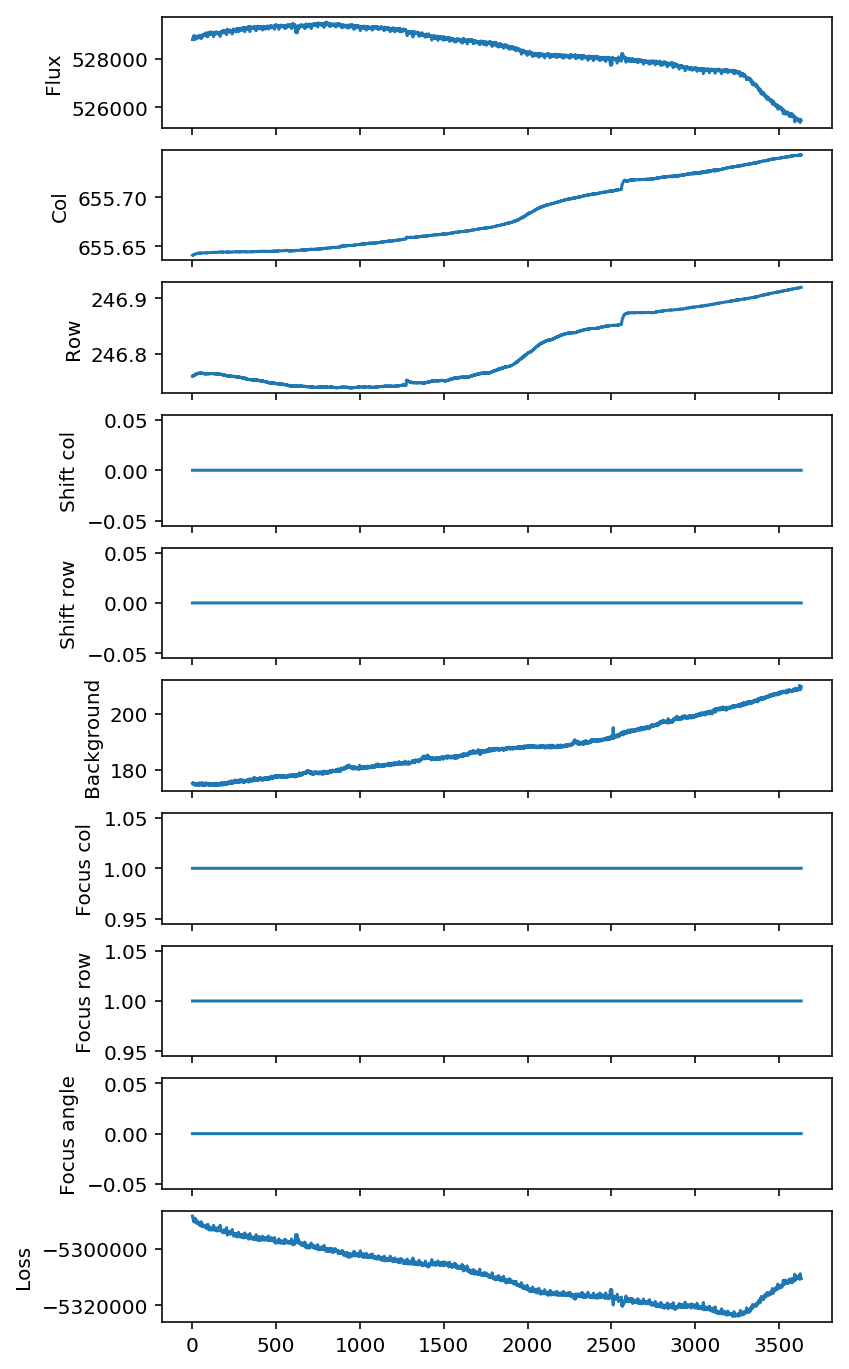You can access the details of the fit in each cadence using the prfphot.results property, e.g.:

In :
prfphot.results.stars.flux
Out:
528783.6252248927

Alternative, you can access the fitted fluxes as a LightCurve object:

In :
prfphot.lightcurves
Out:
<lightkurve.lightcurve.LightCurve at 0x1c24883278>

Note that the focus and motion parameters in the example above are constant. This is because the fit_focus and fit_motion parameters were set to False in the model. By setting these to True, we can potentially improve the quality of the fit. Beware however, this comes at the cost of slowing down the fitting process and potentially introducing more complicated systematics and parameter degeneracies.

In :
prfphot.model.fit_focus = True
prfphot.model.fit_motion = True
prfphot.run(tpf.flux + tpf.flux_bkg)
prfphot.plot_results()
Fitting cadences: 100%|██████████| 3634/3634 [02:17<00:00, 26.47it/s]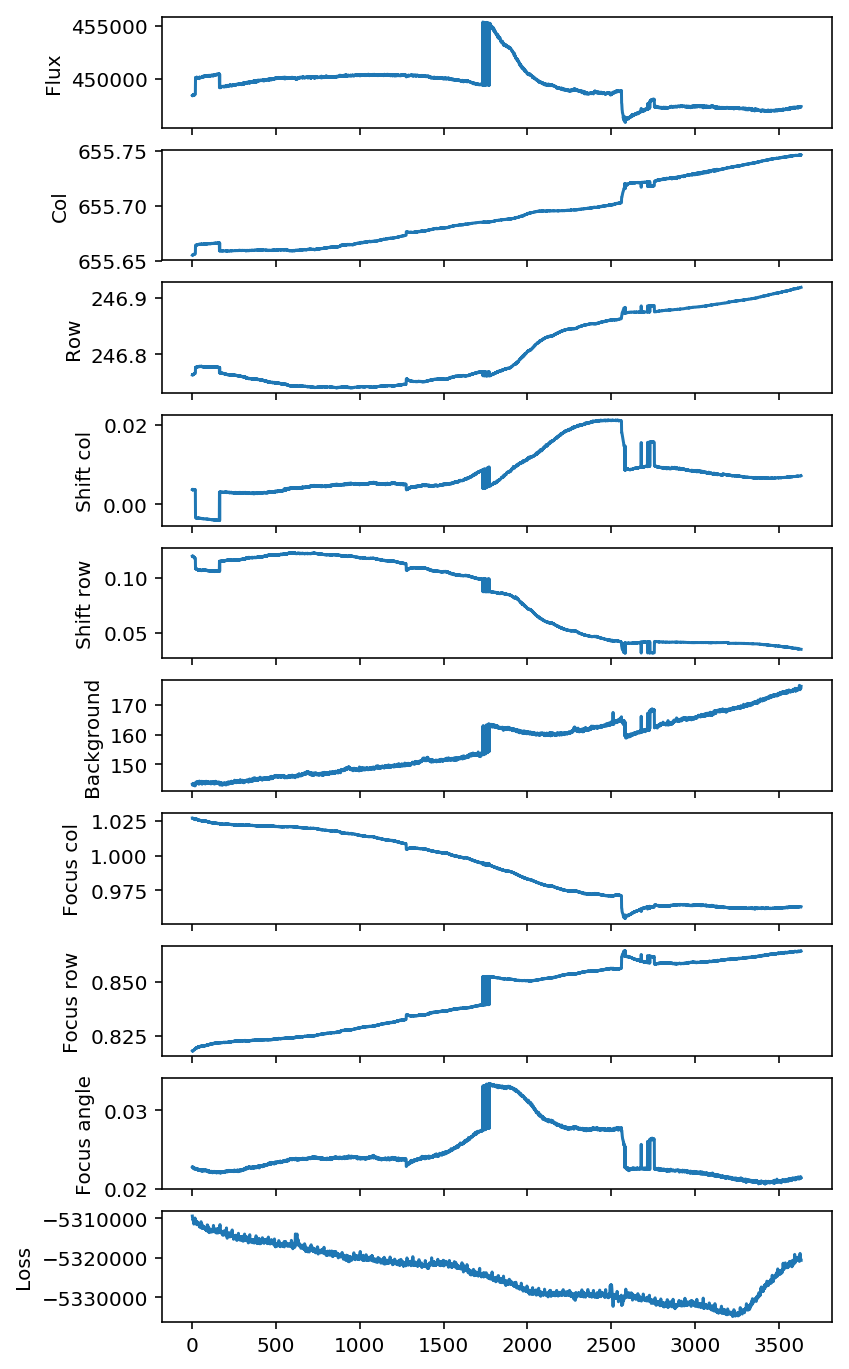In :
prfphot.lightcurves.plot()
Out:
<matplotlib.axes._subplots.AxesSubplot at 0x1c249a39e8>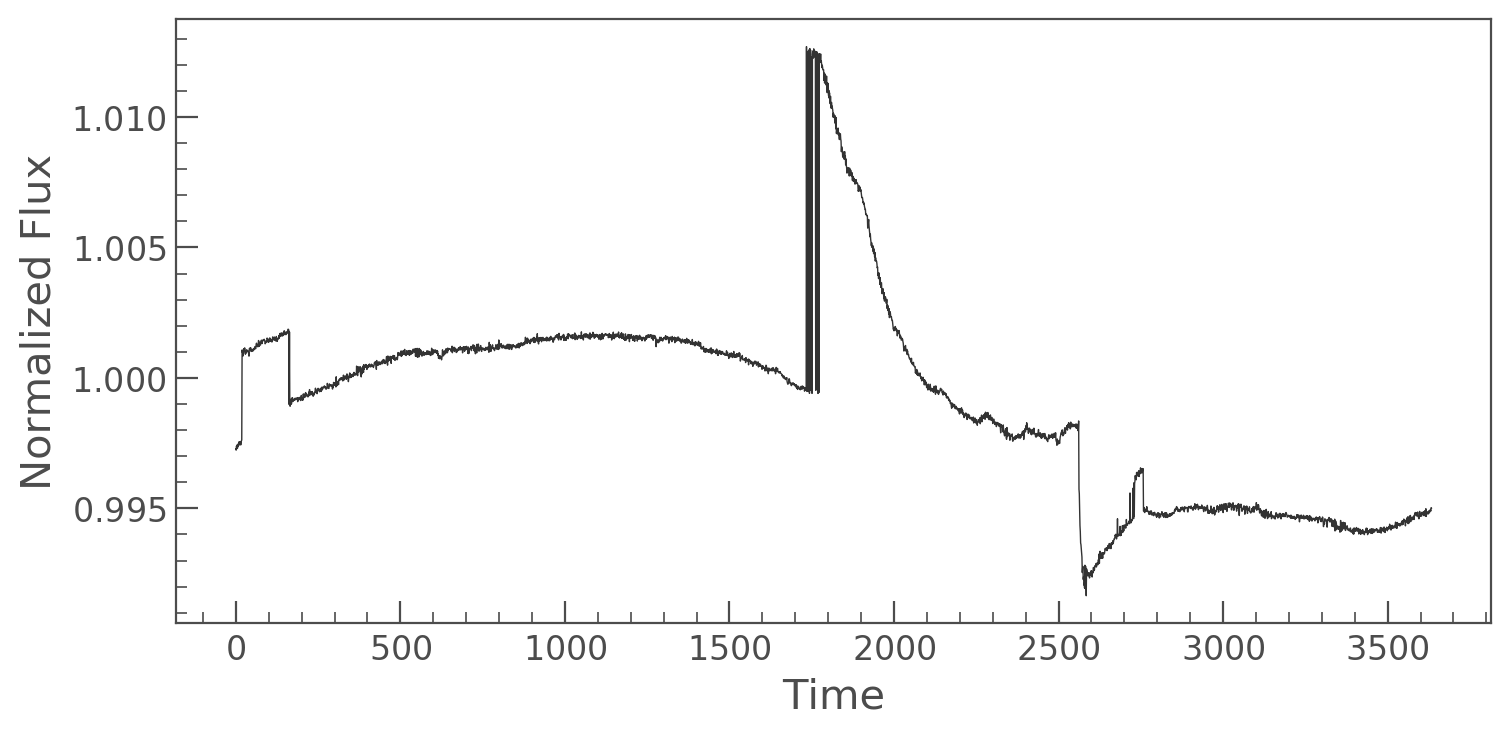Uhoh, the increased model flexibility introduced complicated systematics. These systematics are caused in part by the degeneracy between the star's col and row position and the shift_col and shift_row parameters. The degeneracy will disappear when additional stars are present in the data, or when tighter priors are employed.